MORE IN Elements of Civil Engg. & Engg. Mechanics
VTU First Year Engineering (P Cycle) (Semester 1)
Elements of Civil Engg. & Engg. Mechanics
December 2011
Total marks: --
Total time: --
INSTRUCTIONS
(1) Assume appropriate data and state your reasons
(2) Marks are given to the right of every question
(3) Draw neat diagrams wherever necessary

1 (a) (i) Abuttment is a part of
(B) Bridge
(C) Dam
(D) Building
1 M
1 (a) (ii) Which of the following is not an irrigation infrastructure?
(A) Dam
(B) Canal
(C) Jackwell
1 M
1 (a) (iii) Surveying mainly deals with
(A) Communication
(B) Environment
(C) Material
(D) Measurement
1 M
1 (a) (iv) Geotechnical engineering mainly deals with
(A) Space
(B) Air
(C) Earth
(D) Water
1 M
1 (b) What are the purpose of dam? Name any four types of dams.
8 M
1 (c) Name: (i) Types of roads
(ii) Types of bridges
8 M

2 (a) (i) Two forces having the same line of action are called
(A) Coplanar parallel forces
(B) Non coplanar concurrent forces
(C) Coplanar non concurrent forces
(D) Collinear forces
1 M
2 (a) (ii) The magnitude of the moment is zero, when the force is applied _____ the lever.
(A) Perpendicular to
(B) Inline with
(C) At any angle to
(D) at 60° to
1 M
2 (a) (iii) Following is the unit of moment of a foce
(A) N
(B) Nm2
(C) N2m
(D) Nm
1 M
2 (a) (iv) If two forces are parallel, then they cannot be
(A) Coplanar
(B) Concurrent
(C) Non coplanar
(D) Non concurrent
1 M
2 (b) A block of weight 200N is kept on the inclined plane and is fixed to the plane. Find the component of weight in the direction along the plane and perpendicular to the planes as indicated (refer fig. Q2(b) )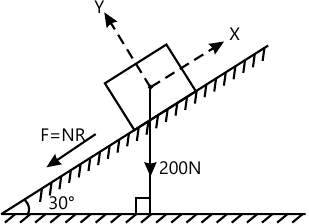4 M
2 (c) Repalce the force system showing in fig. Q2(c) by a single force passing through A and moment of a couple.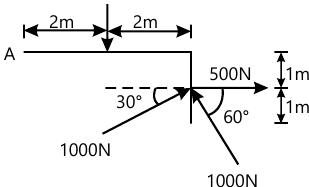6 M
2 (d) State Newton's laws of motion.
6 M

3 (a) (i) The resultant of two concurrent forces becomes minimum if angle between them is _____
(A) Zero
(B) 180°
(C) 90°
(D) 60°
1 M
3 (a) (ii) IF two concurrent forces each of magnitude P act at right angles to each other, their resultant is
(A) 2P
(B) Zero
(C) P√2
(D) (P/2)
1 M
3 (a) (iii) The magnitudes of two given forces are 40N and 60N. Which of the following cannot be their resultant?
(A) 20N
(B) 30N
(C) 40N
(D) 120N
1 M
3 (a) (iv) If the magnitude of resultant of two forces, of each magnitude P, is P, then the angle between the two forces is
(A) Zero
(B) 45°
(C) 120°
(D) 60°
1 M
3 (b) Compute the resultant of the forces, (Refer Fig.Q3(b) )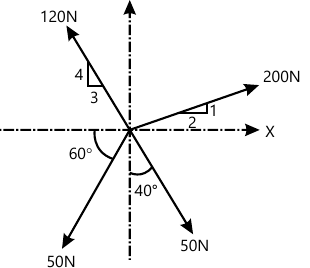8 M
3 (c) The three forces and a moment are applied to a bracket as shown in fig. Q3(c). Determine the moment, M, if the line of action of the resultant of the forces is to pass through B. Compute the resultant of the three forces and the moment.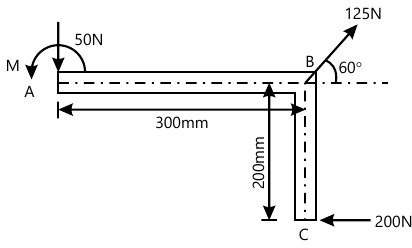8 M

4 (a) (i) Moment of total area about is centroidal axis is ______
(A) Twice the area
(B) Three times the area
(C) Zero
(D) Area x (centroidal distance)2
1 M
4 (a) (ii) For a steel ball of radius, R, ______
(A) The centroidal and centre of gravity are different
(B) The centroidal and centre of gravity are same
(C) The centroidal is half the centre of gravity
(D) None of these
1 M
4 (a) (iii) The co-ordinates of the centroidal of a quadrant of a circle of radius, r is
$(A)\ \bar{x}=\dfrac{4r}{3 \pi},\ \bar{y}=r \\(B)\ \bar{x}=r, \ \bar{y}=\dfrac {4r}{3\pi}\\(C)\ \bar{x}=\dfrac {4r}{3\pi}, \ \bar{y}=\dfrac {4r}{3\pi}\\(D)\ \bar{x}=r, \ \bar{y}=r$
1 M
4 (a) (iv) If the given plane figure is symmetrical about y-y axis only, then the centroid lies on ______
(A) The intersection of x-x axis and y-y axis
(B) x-x axis
(C) y-y axis
(D) None of these
1 M
4 (b) Determine the centroid of a semi circular area of radius r using method of intergration.
8 M
4 (c) Locate the centroid of the shaded area. (All dimensions are in mm refer fig. Q4(c) )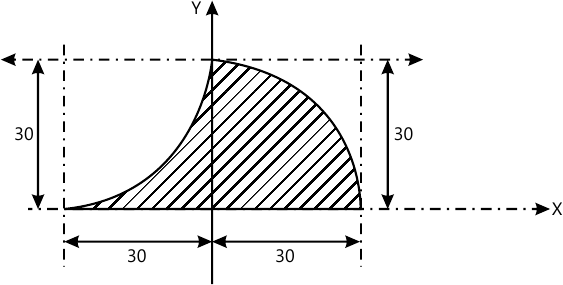8 M

5 (a) (i) A particle acted upon by two forces of equal magnitude having the same line of actio is in equilibrium. The angle between the two forces is _____
(A) 0°
(B) 90°
(C) 180°
(D) 45°
1 M
5 (a) (ii) For equilibrium of a body subjected to coplanar non concurrent forces, the _____
(A) ΣFx=0 and ΣFy=0
(B) ΣFx=2 and ΣM=0
(C) Σm=0
(D) ΣFx=0, ΣFy=0 and Σm=0
1 M
5 (a) (iii) Lami's theorem can be applied when ______ forces act on a body in equilibrium
(A) Two
(B) Three
(C) Four
(D) None of these
1 M
5 (a) (iv) A block of weight, W, is kept on a frictionless inclined plane making an angle, θ with the horizontal. The horizontal forces, P, required to keep block in equilibrium is
(A) W sin θ
(B) (W/2) tan θ
(C) W tan θ
(D) (W/tan θ)
1 M
5 (b) The collar of weight 264.6N may slide on a frictionless vertical rod and is connected to a 294N counter weight, C. Determine the value of 'h' for which the system is in equilibrium (Refer Fig. Q5(b) )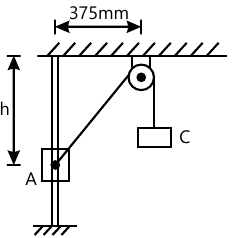6 M
5 (c) Find the force, F action on the crank for equilibrium and also find the reaction at support. Refer Fig.Q5(c) both arms of the crank are of 250 mm length.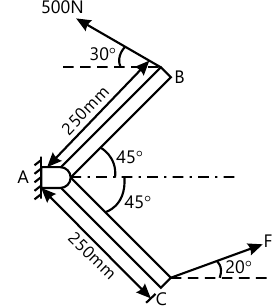10 M

6 (a) (i) For a beam, if one end is supported on roller and the other on hinge, the beam is said to be
(A) Fixed
(B) Hinged
(C) Cantilever
(D) Simply supported
1 M
6 (a) (ii) For a fixed end of a beam, the number of reaction components are ______
(A) Three
(B) Two
(C) One
(D) Zero
1 M
6 (a) (iii) A cantilever beam is one in which _______
(A) Both ends are fixed
(B) One ends is fixed and other is free
(C) Both ends are hinged
(D) Both ends are free
1 M
6 (a) (iv) A horizontal simply supported beam AB of length 5m is acted upon by a vertical point load of 10kN at a distance of 2m from A. The reactions of A and B respectively are
(A) 4kN and 6kN
(B) 6kN and 4kN
(C) 5kN and 5kN
(D) 10kN and Zero
1 M
6 (b) Calculate the reactions at A, for the beam shown in Fig. Q6(b). The beam is hinged at A and supported by cable at C. Self weight of the beam is 2N/m (udl) as indicated.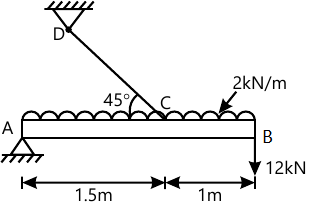6 M
6 (c) For the beam shown in Fig.Q6(c), calculate the ractions at the supports, (Hinged supports at A and support at B)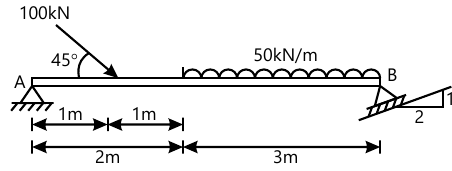10 M

7 (a) (i) Angle of friction is angle between ______
(A) The incline and horizontal
(B) The normal reaction and friction force
(C) The weight of the body and friction force
(D) Normal raction and resultant
1 M
7 (a) (ii) The force of friction depends upon ______
(A) Area of contact
(B) Roughness of surface
(C) Both area of contact and roughness of surface
(D) None of these
1 M
7 (a) (iii) Compared to static friction, kinetic friction is ______
(A) Greater
(B) Smaller
(C) Zero
(D) Very large
1 M
7 (a) (iv) If θ is the angle of friction and α is the angle of repose then which relation is correct ?
(A) θ = 1/α
(B) θ=α
(C) θ = tanα
(D) α = tan θ
1 M
7 (b) The position of the machine block B is adjusted by moving the wedge A. Knowing that the coefficient of static friction is 0.35 between all surface of contact, determine the force, P required to raise the block. B neglect the wieght of wedge. (Refer Fig. Q7(b) ). Weight of block B is 2kN.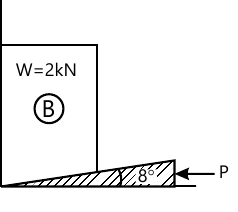10 M
7 (c) A ladder of 4m weighing 200N is supported by a horizontal floor and vertical wall as shown in fig. Q7(c).if an man of weight 650N climbs to the top of the ladder, determine the indication of the ladder with referece of the floor at which the ladder is to placed to prevent slipping. Take the co-efficient of friction for all surface of contact as 0.25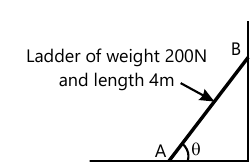6 M

8 (a) (i) The moment of inertia of a circle of diameter D about its centroidal axis is ______
(A) πD2/32
(B) πD2/64
(C) πD4/32
(D) πD4/64
1 M
8 (a) (ii) Moment of inertia is a ______
(A) First moment of area
(B) Second moment of area
(C) Third moment of area
(D) None of these
1 M
8 (a) (iii) Polar moment of inertia of a plane area is ______
(A) Ixx × Iyy
(B) Ixx + Iyy
(C) Ixx / Iyy
(D) None of these
1 M
8 (a) (iv) The unit of moment of inertia of an area is _______
(A) m2
(B) m
(C) m4
(D) m3
1 M
8 (b) State and prove parallel axis theorem :
6 M
8 (c) Find the moment of inertia of plane lamina (shaded) shown if Fig.Q8(c) about x-x axis as indicated.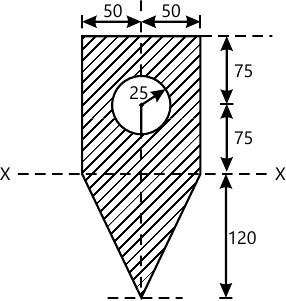10 M

More question papers from Elements of Civil Engg. & Engg. Mechanics